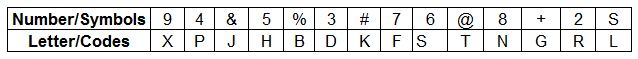# IBPS Clerk 15-Dec-2012

Instructions

Study the following information to answer the given questions
Seven friends — L, M. N, 0. P, Q and R are sitting in a straight line facing North, not necessarily in the same order. M sits fifth to the right of 0. P sits third to the right of L. Both L and P do not sit at the extreme ends of the line. Q and R are immediate neighbours of each other. N sits third to the left of Q.

Question 181

Question 182

Question 183

Question 184

Question 185

# Four of the following live :ire alike in a certain way based on their seating positions in die above arrangement and so form a group. Which is the one that does not belong to the group ?

Instructions

In each question below is given a group of numbers/symbols followed by five combinations of letter codes numbered a: , b:.c:. d: and e:. You have to find out which of the combinations correctly represents the group of numbers/symbols based on the following coding system and the conditions and mark the number of that combination as your answer.Conditions :
(i) If the first element is a symbol and the last element is a number, then the codes for both are to be interchanged.
(ii) If both the first and last elements are symbols, then the last element is to be coded as the code lor the first element.
(iii) If the group of elements contains only one symbol, then that symbols is to be coded as A.

Question 186

Question 187

Question 188

# +5963%

Instructions

Study the following information carefully and answer the questions given below :
Poverty measurement is an unsettled issue, both conceptually and methodologically. Since poverty is a process as well as an outcome: many come out of it while others may be falling into it. The net effect of these two parallel processes is a proportion commonly identified as the ‘head count ratio’, but these ratios hide the fundamental dynamism that characterizes poverty in practice. The most recent poverty re estimates by an expert group has also missed the crucial dynamism. In a study conducted on 13.000 households which represented the entire country in 199394 and again on 2004-05, it was found that in the ten-year period 18.2% rural population moved out of poverty whereas another 22.1% Cell into it over this period. This net increase of about four percentage points was seen to have a considerable variation across states and regions.

Question 189

Question 190

OR